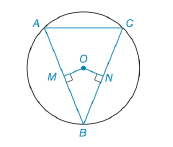Chapter 6.3, Problem 28EElementary Geometry For College St...

7th Edition
Alexander + 2 others
ISBN: 9781337614085

Solutions

Chapter
SectionElementary Geometry For College St...

7th Edition
Alexander + 2 others
ISBN: 9781337614085
Textbook Problem

In Exercises 27 to 30, provide a paragraph proof.Given: ⊙ O   with O M ¯ ⊥ A B ¯     a n d     O N ¯ ⊥ B C ¯ O M ¯ ≅ O N ¯ Secant A C   ¯   t o     ⊙ O Secant A E   ¯   t o     ⊙ Q Prove: Δ A B C is isosceles.To determine

To find:

To prove ΔABC is isosceles.

Explanation

Given that, O with OM¯AB¯andON¯BC¯ and OM¯ON¯.

To prove ΔABC is isosceles that is an isosceles triangle is a triangle that has two sides of equal length.

The diagrammatic representation is given below,

First compare the ΔONB,ΔOMB

But OB=OB. Since its common for both triangles and identity.

Given that OM¯AB¯andON¯BC¯

Therefore, OMB=ONB=90

(SAS) Definition: Triangles are congruent if any pair of corresponding sides and their included angles are equal in both triangles.

So by using side angle side property ΔOMBΔONB.

Also using CPCTC (corresponding parts of congruent triangles are congruent) to get the following,

MB¯=NB¯...(1)

Theorem 6.3.1:

If a line is drawn through the center of a circle perpendicular to a chord, then it bisects the chord and its arc.

That is for circle O given OM¯AB¯, therefore AM¯=MB¯...(2)

Still sussing out bartleby?

Check out a sample textbook solution.

See a sample solution

The Solution to Your Study Problems

Bartleby provides explanations to thousands of textbook problems written by our experts, many with advanced degrees!

Get Started

Solve the differential equation. 13. t2dydt+3ty=1+t2, t 0

Single Variable Calculus: Early Transcendentals

Evaluate 6 8 24 30

Study Guide for Stewart's Multivariable Calculus, 8th

limh0ah1h=1 for a = a) 0 b) 1 c) e d) The limit is never 1.

Study Guide for Stewart's Single Variable Calculus: Early Transcendentals, 8th